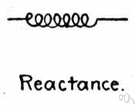# reactance

(redirected from inductive resistance)
Also found in: Thesaurus, Medical, Encyclopedia.
Related to inductive resistance: Inductive reactance

## re·ac·tance

(rē-ăk′təns)
n. Symbol XElectricity
Opposition to the flow of alternating current caused by the inductance and capacitance in a circuit rather than by resistance.

## reactance

(rɪˈæktəns)
n
1. (Electronics) the opposition to the flow of alternating current by the capacitance or inductance of an electrical circuit; the imaginary part of the impedance Z, Z = R + iX, where R is the resistance, i = √–1, and X is the reactance. It is expressed in ohms. Compare resistance3
2. (General Physics) the opposition to the flow of an acoustic or mechanical vibration, usually due to inertia or stiffness. It is the magnitude of the imaginary part of the acoustic or mechanical impedance
Collins English Dictionary – Complete and Unabridged, 12th Edition 2014 © HarperCollins Publishers 1991, 1994, 1998, 2000, 2003, 2006, 2007, 2009, 2011, 2014

## re•ac•tance

(riˈæk təns)

n.
the opposition of inductance and capacitance to alternating electrical current, expressed in ohms. Symbol: X
 Noun 1reactance - opposition to the flow of electric current resulting from inductance and capacitance (rather than resistance)electrical phenomenon - a physical phenomenon involving electricity